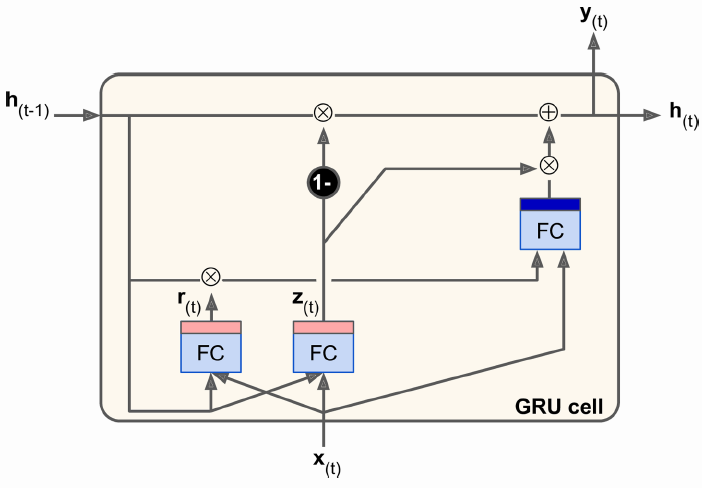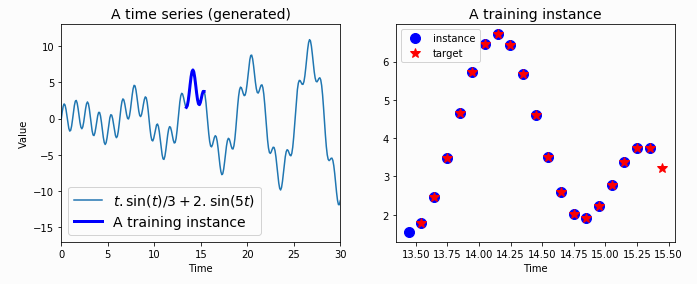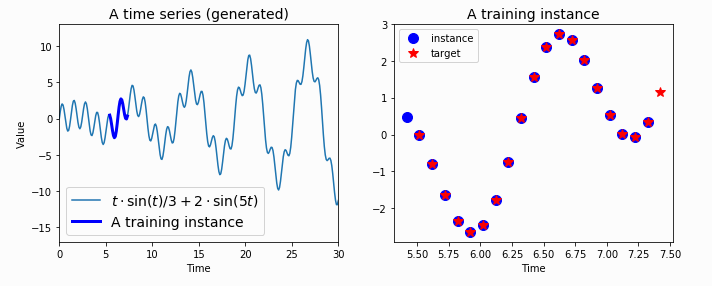## 2019/1/23

### Recurrent Neural Networks (RNN) 原理 2/2

28 Recurrent Neural Networks (RNN) 原理 2/2

► Long Short-Term Memory (LSTM) cell
Long Short-Term Memory (LSTM) 中文可說成長短項記憶 ，一個 LSTM 晶元內部有兩個記憶的狀態，一為長項記憶，一為短項記憶，故有此名稱。想像一般的 RNN cell ，若所需處理的時階很長，如50 ，則開始後的第一個時階 的記憶傳遞到最後一個時階會逼近0 （想像$0.9^{50}\cong 0.0051$），所以對長時階的處理會有困難，這就是記憶消失的問題。為了解決此一問題，有人就提出各種不同的策略，LSTM 以及其各種變型是比較受歡迎的一種。在一般的LSTM 中的短記憶就等同一般 RNN cell 裡的隱藏狀態的記憶，此記憶不能傳遞於太長的時階，故稱短記憶，另有一種可於長時階傳遞、不易衰減的記憶稱為長記憶，此記憶狀態就是用來改善記憶消失的問題。LSTM cell

◆ forget gate （遺忘閘）

$\textbf{f}_{(t)}=\sigma \left ( \textbf{W}_{xf} ^T \cdot\textbf{x}_{(t)} + \textbf{W}_{hf} ^T \cdot\textbf{h}_{(t-1)} +\textbf{b}_f\right )$

◆ input gate （輸入閘）

$\textbf{i}_{(t)}=\sigma \left ( \textbf{W}_{xi} ^T \cdot\textbf{x}_{(t)} + \textbf{W}_{hi} ^T \cdot\textbf{h}_{(t-1)} +\textbf{b}_i\right )$
$\textbf{g}_{(t)}=\tanh \left ( \textbf{W}_{xg} ^T \cdot\textbf{x}_{(t)} + \textbf{W}_{hg} ^T \cdot\textbf{h}_{(t-1)} +\textbf{b}_g\right )$

◆ output gate （輸出閘）

$\textbf{o}_{(t)}=\sigma \left ( \textbf{W}_{xo} ^T \cdot\textbf{x}_{(t)} + \textbf{W}_{ho} ^T \cdot\textbf{h}_{(t-1)} +\textbf{b}_o\right )$

◆ output functions （輸出函數）
LSTM cell 有三個輸出，分別為
$\textbf{c}_{(t)}=\textbf{c}_{(t-1)}\otimes \textbf{f}_{(t)}+\textbf{g}_{(t)}\otimes \textbf{i}_{(t)}$
$\textbf{h}_{(t)}=\textbf{o}_{(t)}\otimes \tanh \left ( \textbf{c}_{(t)} \right )$
$\textbf{y}_{(t)}=\phi \left ( \textbf{W}_{hy} ^T \cdot\textbf{h}_{(t)} +\textbf{b}_y\right )$

◆ Peephole Connections （窺孔連接）

► Gated Recurrent Unit (GRU) cell
GRU 的中文可說成閘控遞迴單元，是一種受歡迎的 LSTM cell 的變型，其構造如下圖所示。GRU cell

• 長記憶和短記憶兩個狀態向量被合併為1，稱$\textbf{h}_{(t)}$，這又回到基本 RNN cell 的一個記憶狀態。
• forget gate 和 input gate 由一函數控制。如果此控制函數的某元素是1，則 input gate 對應的元素打開，但 forget gate 則是關閉，如果輸出是0，則作用相反。
• 並無output gate, 記憶向量直接輸出至下一時階，但另有一個新的控制閘用以控制記憶向量的那些部份要和輸入訊號一起加到此層的主要輸出操作。

$\textbf{z}_{(t)}=\sigma \left ( \textbf{W}_{xz} ^T \cdot\textbf{x}_{(t)} + \textbf{W}_{hz} ^T \cdot\textbf{h}_{(t-1)} +\textbf{b}_z \right )$
$\textbf{r}_{(t)}=\sigma \left ( \textbf{W}_{xr} ^T \cdot\textbf{x}_{(t)} + \textbf{W}_{hr} ^T \cdot\textbf{h}_{(t-1)} +\textbf{b}_r \right )$
$\textbf{g}_{(t)}=\tanh \left ( \textbf{W}_{xg} ^T \cdot\textbf{x}_{(t)} + \textbf{W}_{hg} ^T \cdot \left ( \textbf{r}_{(t)} \otimes \textbf{h}_{(t-1)} \right ) +\textbf{b}_g \right )$
$\textbf{h}_{(t)}=\left ( 1- \textbf{z}_{(t)}\right )\otimes \tanh \left ( \textbf{W}_{xg} ^T \cdot\textbf{h}_{(t-1)} +\textbf{z}_{(t)}\otimes \textbf{g}_{(t)}\right )$

► TensorFlow tf.nn.rnn_cell 模組

• BasicRNNCell: 這是個最基本的RNN cell，但已被棄置(Deprecated) 。官網說明其功能等效於 tf.keras.layers.SimpleRNNCell, 於 Tensorflow 2.0 版中會被取代。
• BasicLSTMCell: 也是被被棄置，官網說明請用  tf.nn.rnn_cell.LSTMCell 代替。
• LSTMCell: 長短期記憶 cell。
• GRUCell: Gated Recurrent Unit cell。
• RNNCell: 用抽象物件表示一個 RNN cell.
• MultiRNNCell: 依順序串接多個簡單的 cells.
◆ LSTMCell:

__init__(
num_units,
use_peepholes=False,
cell_clip=None,
initializer=None,
num_proj=None,
proj_clip=None,
num_unit_shards=None,
num_proj_shards=None,
forget_bias=1.0,
state_is_tuple=True,
activation=None,
reuse=None,
name=None,
dtype=None,
**kwargs
)


• num_units: 要產生cells的數目
• use_peepholes=False，預設False ，可改成True
• cell_clip=None, 設輸出值的最高限制，超過則砍掉。
• forget_bias=1.0, 遺忘閘初值，設1.0 是表示全部通過，如果初始時沒設1.0 則可能一開始時長記憶狀態就通過不了。
• activation=None, 作動函數預設是None，需要時可加入。
◆ GRUCell:

__init__(
num_units,
activation=None,
reuse=None,
kernel_initializer=None,
bias_initializer=None,
name=None,
dtype=None,
**kwargs
)


• num_units: 要產生cells的數目
• activation=None, 作動函數預設是None，需要時可加入。
• bias_initializer=None,預設偏置項不初始化
• reuse=None，相同名稱的cell 不重複使用。
◆ tf.keras.layers.SimpleRNNCell

__init__(
units,
activation='tanh',
use_bias=True,
kernel_initializer='glorot_uniform',
recurrent_initializer='orthogonal',
bias_initializer='zeros',
kernel_regularizer=None,
recurrent_regularizer=None,
bias_regularizer=None,
kernel_constraint=None,
recurrent_constraint=None,
bias_constraint=None,
dropout=0.0,
recurrent_dropout=0.0,
**kwargs
)


• units, 要產生cells的數目 ，注意在tf.nn.LSTMCell 和GRUCell中使用的是num_units，不知為要設不樣！
• activation='tanh', 預設是用tanh。
• use_bias=True, 預設是True
• bias_initializer='zeros', 預設是0。
► tf.nn.static_rnn

tf.nn.static_rnn(
cell,
inputs,
initial_state=None,
dtype=None,
sequence_length=None,
scope=None
)


• cell: RNNCell class 的一個實例
• inputs: 一個長度為 T 的list 輸入, Tensor 的外形為 [batch_size, input_size], 或是這種元素的巢狀tuple。
• initial_state:一個選用的RNN 初始狀態。. 如果狀態的尺度是一整數，那必需是一個tensor 具適當的型態和外形 [batch_size, cell.state_size].如果cell 的 state_size 是一個 tuple, 那應是一個tensors 的tuple 具有外形 [batch_size, s]  其中s 在 state_size裡。
• type: (選用)初始狀態和期望輸出的data type . 當 initial_state 未提供 或  RNN 狀態的 dtype 具相異類型。
• sequence_length:序列長度，指定在輸入裡每序列的長度為一 int32 或 int64 的 vector (tensor) 尺度為 [batch_size], v其值在 [0, T) 之間。
• scope:  產生子圖的VariableScope;預設為"rnn".
• 傳回 (outputs, state) ，其中 outputs是長度為 T的 list  (每一輸入一個), 這種元素的巢狀tuple。
► tf.nn.dynamic_rnn 這是個動態的method，一樣是用以建立參數cell 指定的RNN 網路，但是不像靜態cell 方法於編譯時就建立，動態cell 是於run-time 才建立。其參數如下
tf.nn.dynamic_rnn(
cell,
inputs,
sequence_length=None,
initial_state=None,
dtype=None,
parallel_iterations=None,
swap_memory=False,
time_major=False,
scope=None
)


• cell: RNNCell class 的一個實例
• inputs: RNN 的輸入，一般是 [None, time_step, features] ，這是當 time_major == False (預設), 也可以是這樣元素的巢狀tuple。如果 time_major == True, 則輸入必需是外形為 [max_time, batch_size, ...], 或者是這樣元素的巢狀 tuple. 一個 Tensors  的 tuple   (可能是巢狀) 也可偶滿足此性質。前兩維必需和所有輸入匹配，但秩或其他外形成份可能不同  此情形每一時階饋到cell 的輸入將複製這些tuples 的結構，除時間維（由此取出時間）之外。每一時階饋到cell 的輸入為一 Tensor 或是  Tensors 的 tuple (可為巢狀) 個別的維度為 [batch_size, ...].
• sequence_length: (選用) 序列長，為一 int32/int64 vector 不度為  [batch_size]。當傳遞匹量元素的序列長度時用於複製 state and zero-out 輸出，所以用於性能比用於正確性成份多。
• initial_state: (選用) 初始狀態， RNN 的初始狀態，如果狀態的尺度是一整數，那必需是一個tensor 具適當的型態和外形 [batch_size, cell.state_size].如果cell 的 state_size 是一個 tuple, 那應是一個tensors 的tuple 具有外形 [batch_size, s]  其中s 在 state_size裡。
• type: (選用)初始狀態和期望輸出的data type . 當 initial_state 未提供 或  RNN 狀態的 dtype 具相異類型。
• parallel_iterations: (預設 32). 併行運行的迭代數目，可用於沒有任何暫時相依，可平行運行的操作。此參數以空間交換時間，此值Values >> 1 使用更多記憶體但省時間，而較小的值使用較少記憶體但計算較久。
• swap_memory: 交換記憶體，透通地交換在訓向推論產生的Tensor 但需由GPU 到 CPU 後向傳播，這允許訓練典型不能於單一GPU 調適的 RNNs ，具有很小（或沒有）性能懲罰。
• time_major: 時間為主，是一種輸入和輸出Tensor 的外形格式。若設為True, 則這些tensor 的外形必需是 [max_time, batch_size, depth]. 若設為False,則這些tensor 的外形必需是[batch_size, max_time, depth]. 使用 time_major = True 有點較有效率，因如此避免在RNN 計算的開始和結束時轉置。然而，大部份的TensorFlow 的資料是匹量為主(batch-major), 所以此函數預設是False，亦即以匹量為主的格式接受輸入和饋送輸出。
• scope:  產生子圖的VariableScope;預設為"rnn"
• 傳回 (outputs, state) ，其中 outputs是此 RNN 的輸出Tensor。如果使用設的 time_major == False ，則Tensor的外形為 [batch_size, max_time, cell.output_size]. 如果設 time_major == True, 則Tensor的外形為 shaped: [max_time, batch_size, cell.output_size].  state: 是最後的狀態， 如果 cell.state_size 是整數,則外形為 [batch_size, cell.state_size]。如果是一 TensorShape 則外形為[batch_size] + cell.state_size. 如果是ints or TensorShape (可能巢狀） 則為一有相當外形的tuple，如果cell 是 LSTMCells 則 state 將是一個 tuple 含每一cell 的 LSTMStateTuple 。
► tf.contrib.rnn.OutputProjectionWrapper

__init__(
cell,
output_size,
activation=None,
reuse=None
)


• cell: 一 RNNCell, 加上投影到輸出的尺度。
• output_size: 輸出尺度，為一整數 ，在投影之後的輸出尺度。
• activation: (選用) 作動函數，不用就是線性。
• reuse: (選用) 描述是否在所在的範圍內重用變數的Python 布林變數。如果不是設為 If not True, 而在所在範圍內有相同名稱的變數將產生錯誤。
► tf.contrib.rnn.OutputProjectionWrapper

1. 定義參數，如batch size, num_unit, n_step , features等。
2. 宣告要使用cell 的實例，如 simple cell, LSTM cell, GRU cell 等。
3. 叫用tf.nn.dynamic_rnn  method,
4. 定義loss function，如  xentropy
5. 定義優化器，並代入loss function
6. 建立sess，重覆饋入訓練集進行訓練
7. 饋入測集求導 model 性能。
►產生時間序列資料 除Natural Language Processing (自然語言處理，NLP) ，Time Series Analysis (時間序列分析，TSA) 也是RNN 很適合的應用領域，但要練習程式最簡單的方法就是產生要訓練的data，但如果相鄰時間的資料都是隨機產生則預測就沒有意義，所以我們必需產生時間上相關的訊號讓RNN 學習並預測才有意義。所以在討論實例之前，我們就先來看看如何產生時間序列的資料。

$t \cdot \sin(t) / 3 + 2 \cdot \sin(5t)$

import numpy as np
import tensorflow as tf
import matplotlib.pyplot as plt
# %matplotlib inline
t_min, t_max = 0, 30
resolution = 0.1
def time_series(t):
return t * np.sin(t) / 3 + 2 * np.sin(t*5)
batch = 20
pitch = batch +  1
t = np.linspace(t_min, t_max, int((t_max - t_min) / resolution))
series = time_series(t)

kk = np.random.randint(300-pitch)
tx_data = t[kk:kk+batch]
ty_data = t[kk+1:kk+1+batch]
aa = series[kk:kk+pitch]
x_data = aa[0:batch]
y_data = aa[1:pitch]

plt.figure(figsize=(11,4))
plt.subplot(121)
plt.title("A time series (generated)", fontsize=14)
plt.plot(t, time_series(t), label=r"$t \cdot \sin(t) / 3 + 2 \cdot \sin(5t)$")
plt.plot(tx_data, x_data, "b-", linewidth=3, label="A training instance")
plt.legend(loc="lower left", fontsize=14)
plt.axis([0, 30, -17, 13])
plt.xlabel("Time")
plt.ylabel("Value")

plt.subplot(122)
plt.title("A training instance", fontsize=14)
plt.plot(tx_data, x_data, "bo", markersize=10, label="instance")
plt.plot(ty_data, y_data, "r*", markersize=10, label="target")
plt.legend(loc="upper left")
plt.xlabel("Time")
plt.show()時間序列1時間序列2

https://www.tensorflow.org/tutorials/
Aurélien Géron, Hands-On Machine Learning with Scikit-Learn and TensorFlow, O'Reilly, 2017